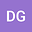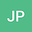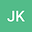Mass conserving global solutions for the nonlinear collision-induced fragmentation model with a singular kernel
•••Jitendra Kumar
Indian Institute of Technology Kharagpur
Author Profile## Abstract

This article is devoted to the study of existence of a mass conserving global solution for the collision-induced nonlinear fragmentation model which arises in particulate processes, with the following type of collision kernel: $C(x,y)~\le~k_1 \frac{(1 + x)^\nu (1 + y)^\nu}{\left(xy\right)^\sigma},$ for all ~$x, y \in (0,\infty)$, where $k_1$ is a positive constant, $\sigma \in \left[0,\tfrac{1}{2}\right]$ and $\nu \in [0, 1]$. The above-mentioned form includes many practical oriented kernels of both \emph{singular} and \emph{non-singular} types. The singularity of the unbounded collision kernel at coordinate axes extends the previous existence result of Paul and Kumar [Mathematical Methods in the Applied Sciences 41 (7) (2018) 2715–2732 (\href{https://doi.org/10.1002/mma.4775}{doi:10.1002/mma.4775})] and also exhibits at most quadratic growth at infinity. Finally, uniqueness of solution is also investigated for pure singular collision rate, i.e., for ~ $\nu=0$.